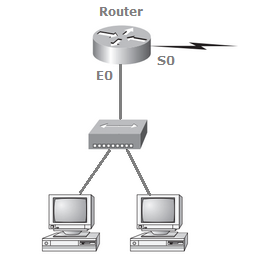# Networking - Subnetting

### Exercise :: Subnetting - Subnetting

• Subnetting - Subnetting
16.

If a host on a network has the address 172.16.45.14/30, what is the subnetwork this host belongs to?

 A. 172.16.45.0 B. 172.16.45.4 C. 172.16.45.8 D. 172.16.45.12

Explanation:

A /30, regardless of the class of address, has a 252 in the fourth octet. This means we have a block size of 4 and our subnets are 0, 4, 8, 12, 16, etc. Address 14 is obviously in the 12 subnet.

17.

Using the following illustration, what would be the IP address of E0 if you were using the eighth subnet? The network ID is 192.168.10.0/28 and you need to use the last available IP address in the range. The zero subnet should not be considered valid for this question.A. 192.168.10.142 B. 192.168.10.66 C. 192.168.100.254 D. 192.168.10.143 E. 192.168.10.126

Explanation:

A /28 is a 255.255.255.240 mask. Let's count to the ninth subnet (we need to find the broadcast address of the eighth subnet, so we need to count to the ninth subnet). Starting at 16 (remember, the question stated that we will not use subnet zero, so we start at 16, not 0), 16, 32, 48, 64, 80, 96, 112, 128, 144. The eighth subnet is 128 and the next subnet is 144, so our broadcast address of the 128 subnet is 143. This makes the host range 129-142. 142 is the last valid host.

18.

Which configuration command must be in effect to allow the use of 8 subnets if the Class C subnet mask is 255.255.255.224?

 A. Router(config)#ip classless B. Router(config)#no ip classful C. Router(config)#ip unnumbered D. Router(config)#ip subnet-zero

Explanation:

A Class C subnet mask of 255.255.255.224 is 3 bits on and 5 bits off (11100000) and provides 8 subnets, each with 30 hosts. However, if the command ip subnet-zero is not used, then only 6 subnets would be available for use.

19.

Using the illustration from the previous question, what would be the IP address of S0 if you were using the first subnet? The network ID is 192.168.10.0/28 and you need to use the last available IP address in the range. Again, the zero subnet should not be considered valid for this question.

 A. 192.168.10.24 B. 192.168.10.62 C. 192.168.10.30 D. 192.168.10.127

Explanation:

A /28 is a 255.255.255.240 mask. The first subnet is 16 (remember that the question stated not to use subnet zero) and the next subnet is 32, so our broadcast address is 31. This makes our host range 17-30. 30 is the last valid host.

20.

What is the subnetwork address for a host with the IP address 200.10.5.68/28?

 A. 200.10.5.56 B. 200.10.5.32 C. 200.10.5.64 D. 200.10.5.0